News Forums RAIN General Discussion and Troubleshooting Pathfinding on dynamic voxel block terrain

Viewing 14 posts - 1 through 14 (of 14 total)
• Author
Posts
• #38822

Hi!

Ill try to explain my problem briefly.

1. Ive a terrain which is consisting of Chunk (GameObject)with a mesh that holds a set of blocks, what basically is a lowest unit in terrain. Those blocks can be created and destroyed at runtime. This terrain is generated at runtime and blocks can be edited at runtime.
Image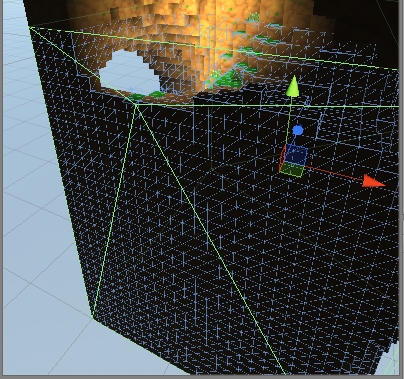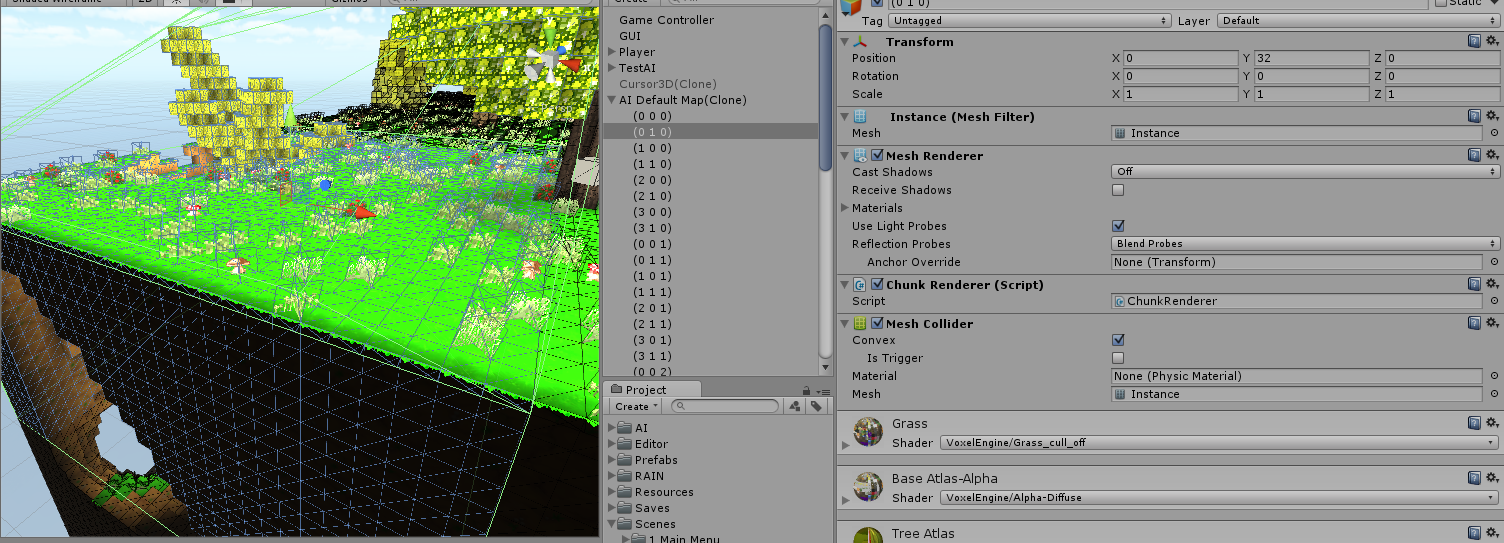As You can probably see - ive added a Convex mesh collider, but it seems that convex wont do the thing here.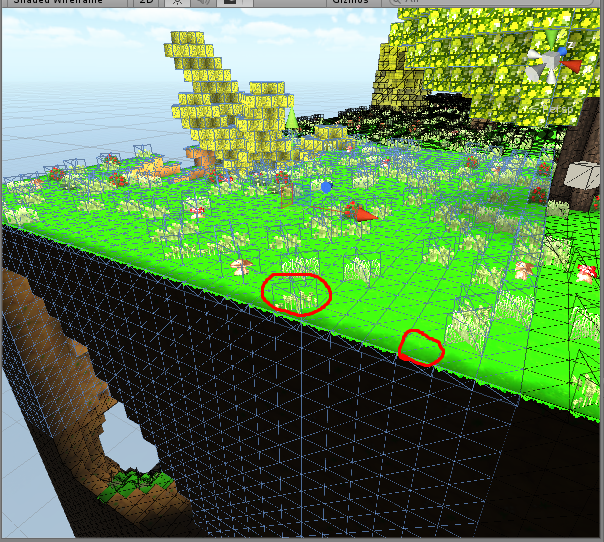Here i have the same chunk with meshcollider, but its not convex anymore. Ive emphasized two blocks with cycles.

2. This terrain uses custom Physics and with all that - no Unity raycast support (custom intersection logic / raycasts). For example, Player and AIs use custom character controller;

3. As Terrain is generated at runtime and can be updated at runtime (each chunk can be changed in realtime) - AFAIK there is no way to actually use RAIN Navmesh for navigation (runtime navmesh gen is not an option because on terrain create it will work, but at runtime chunk updates it would freeze the game as Navmeshes should be re-generated);
For example, i want to suddenly create a hole - AI should be immediately be aware of that and avoid it.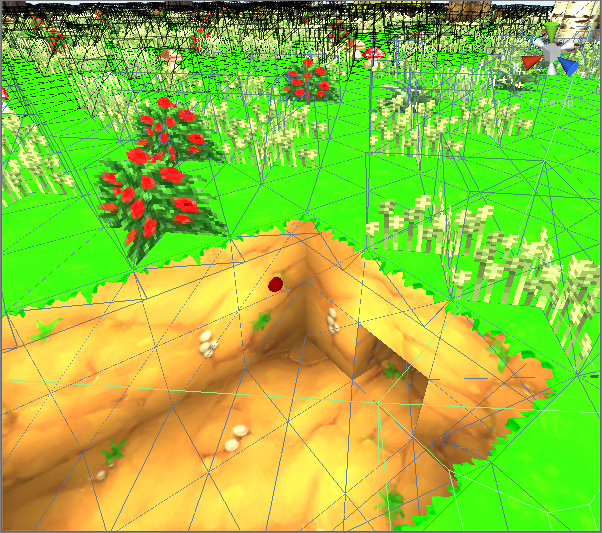4. This Meshcollider that im adding to each chunk is a unused feature - its added specially for RAIN (currently it uses memory for no reason - if there is a way to not use it - i would love it and remove it);

What i have done so far?

1. Added CustomCharacterMotor and managed to use the custom CharacterController that this VoxelEngine uses. See code here -> http://hastebin.com/oludobejak.avrasm
Movement is working fine.
2. Added Custom Navigator, but havent got any functionality working - thats why i am writing here. Current version: http://hastebin.com/poxinirofi.cs

I would love to somehow get AIs to be aware of current Terrain(the same layer where they are)
do i need to create custom pathfinding or somehow register my blocks (they are not gameObjects) for RAIN as obstacles or somehow build a navmesh-like mesh for each chunk that AI uses for pathfinding ?

Any ideas? Sigil, perhaps You can help me out with this.

Please dont hesitate to ask more questions or correct me regarding this problem. I need to lauch this game ASAP.

Best regards,
Sacristan

#38824

The problem here is that Navigator (and many other things) lack documentation.

I pretty much have read through many forum topics - here are few of those:
http://rivaltheory.com/forums/topic/custom-navigator-for-a-pathfinding-project/
http://rivaltheory.com/forums/topic/custom-nav-mesh/
http://rivaltheory.com/forums/topic/destructable-voxel-terrain-and-navmeshes/

Btw, as ill be using WebGL i cant use Threads

#38836

Hi @Sacristan, thanks for coming to the forums,

I know we’ve spoken a couple times (through Jester) about your problem, but I’m glad you’re here as I think we can put together a solution for it, as I have a few ideas (better than the Unity Navigation Mesh one that I mentioned in email).

I’ll look over the code you posted, and see if I can’t put something together. My initial thoughts are this: The only real static content in any RAIN navigation mesh are the vertices, which should line up very well with your voxel data (I’m assuming). After I add a RemoveNode function to the Navigation Mesh, I see no reason why we can’t setup a way to add and remove polygons from the Navigation Mesh as voxels are added and removed from your mesh. I need to look into improving our path smoothing as well though, to handle the movement across polys with lots of interior vertices, but I think that is doable, and necessary anyhow.

I’ll start by setting up some code to add voxel data to a custom navigation mesh and create an initial set of walkable polys.

#38854

Ty for Your awesome response @Sigil!

I have been talking with @Jester mostly, but its a lot better to post a problem here - perhaps someone has something similar.

Sac

#38874

So here is a first pass at something, just to start feeling things out. This script generates a voxel like Navigation Mesh based on the parameters it has. You can put this on a Navigation Mesh and try it out (hit Create Vertices, then Add Polys in the component).

If you leave this in the root of the scene it will create the Navigation Mesh at (0, 0, 0), but if you parent it to a GameObject you can actually move it around. You can kinda see how you could parent one of these to each of your voxel blocks, so that each one has it’s own setup.

This really is just a first pass to start seeing how you can work it into your code. It doesn’t have a way of turning off any of the squares, which I will mess around with next. Try this in a blank scene first just to see how it works, make sure it has a Navigation Mesh on the object too, let me know if there are issues.

```using RAIN.Navigation.NavMesh;
using UnityEngine;
[ExecuteInEditMode]
public class CubeNavMesh : MonoBehaviour
{
[SerializeField]
private bool _createVertices = false;
[SerializeField]
[SerializeField]
private int _cubeCount = 5;
[SerializeField]
private float _cubeSize = 2;
private NavMeshRig _rig = null;
public void Update()
{
if (_rig == null)
_rig = gameObject.GetComponent<NavMeshRig>();
if (_rig == null)
return;
if (_createVertices)
{
_createVertices = false;
// Clear the graph so that we start fresh
// We need one more vertex to handle our cubes properly
int tVertexPerRow = _cubeCount + 1;
int tVertexPerPlane = tVertexPerRow * tVertexPerRow;
// Our Navigation Mesh will be multiple levels of squares
Vector3[] tVertices = new Vector3[tVertexPerRow * tVertexPerRow * tVertexPerRow];
for (int i = 0; i < tVertices.Length; i++)
{
tVertices[i] = new Vector3((i % tVertexPerRow) - (_cubeCount / 2f),
(i / tVertexPerPlane) - (_cubeCount / 2f),
(i / tVertexPerRow) % tVertexPerRow - (_cubeCount / 2f));
tVertices[i] *= _cubeSize;
}
// Editor has to serialize to save
if (!Application.isPlaying)
_rig.Serialize();
}
{
if (_rig.NavMesh.Graph.Vertices.Count == 0)
return;
int tPolyCount = _cubeCount * _cubeCount * _cubeCount;
int tPolyPerPlane = _cubeCount * _cubeCount;
int tVertexPerRow = _cubeCount + 1;
int tVertexPerPlane = tVertexPerRow * tVertexPerRow;
for (int i = 0; i < tPolyCount; i++)
{
int tFirstRow = (i / tPolyPerPlane) * tVertexPerPlane + (i / _cubeCount) % _cubeCount * tVertexPerRow;
int tSecondRow = (i / tPolyPerPlane) * tVertexPerPlane + ((i / _cubeCount) % _cubeCount + 1) * tVertexPerRow;
int[] tPoly = new int[]
{
(i % _cubeCount) + tFirstRow,
(i % _cubeCount) + tSecondRow,
((i % _cubeCount) + 1) + tSecondRow,
((i % _cubeCount) + 1) + tFirstRow,
};
// Our triangle
int[] tTriangles = new int[]
{
(i % _cubeCount) + tFirstRow,
(i % _cubeCount) + tSecondRow,
((i % _cubeCount) + 1) + tSecondRow,
((i % _cubeCount) + 1) + tSecondRow,
((i % _cubeCount) + 1) + tFirstRow,
(i % _cubeCount) + tFirstRow,
};
// Our edges
int[] tEdges = new int[tPoly.Length * 2];
for (int j = 0; j < tEdges.Length; j += 2)
{
tEdges[j] = tPoly[j / 2];
tEdges[j + 1] = tPoly[(j / 2 + 1) % tPoly.Length];
}
// Create our navigation mesh poly
NavMeshPoly tNavMeshPoly = new NavMeshPoly(_rig.NavMesh.Graph, tPoly, tTriangles);
// And create all of our navigation mesh edges
for (int j = 0; j < tEdges.Length; j += 2)
{
NavMeshEdge tNavMeshEdge = new NavMeshEdge(_rig.NavMesh.Graph, tEdges[j], tEdges[j + 1]);
}
// And connect them
tNavMeshPoly.ConnectAllEdges();
}
// Turn our display on
// Editor has to serialize to save
if (!Application.isPlaying)
_rig.Serialize();
}
}
}```
#38875

Great!

Already had some fun with it today. Ill check it in more depth tomorrow.

#38880

It looks like currently this is only Editor time option.

```// Editor has to serialize to save
if (!Application.isPlaying)
_rig.Serialize();```

Ill have to update this navmesh at runtime.

Currently this greatly convers layers

——-
I would need to set up this for every and each of chunk AFAIK. Here i would need to write the cube count and cube size grid, it would be great that a square within square layer grid could be turned off (as You mentioned)

It pretty much have to work on terrain like

Here it can be seen that there is 3, 2 and 1 block depth hole between two even “platforms”. It would be great to ignore these holes during navmesh generation, so when AI needs to get from even platform 1 to even platform 2, it would not go through the hole, but around it.

#38887

As for the option that is editor only, serialization only matters when you are in the editor, so that is why that check is there. If you use it at runtime it will work all the same. I only put the line there so that if you generated the Navigation Mesh while in the editor it would save properly.

So of course, this is just a first pass and is incomplete. What I would like to see is if you can start integrating this into your code and what problems you run into. Can you get it to line up properly with your chunks and blocks?

I’ve already realized there is an issue with the way the Navigation Mesh is generated that will cause problems: it isn’t taking agent size into account. So I’m going to take a look at that while I add in the adding/removing of sections.

No worries though, I’ll be working on this for awhile, I like to see how RAIN is being used and what I can do to improve it.

#38891

Here is an updated version with some AddSquare/RemoveSquare functions. These functions mark sections as unwalkable, but not in a voxel way. This may not work properly for pathing, as the walkable/unwalkable setup doesn’t work quite right with the current Navigation Meshes, I think. Still working on it:

```using RAIN.Navigation.NavMesh;
using UnityEngine;
[ExecuteInEditMode]
public class CubeNavMesh : MonoBehaviour
{
[SerializeField]
private bool _createVertices = false;
[SerializeField]
[SerializeField]
private int _cubeCount = 5;
[SerializeField]
private float _cubeSize = 2;
private NavMeshRig _rig = null;
public void Update()
{
if (_rig == null)
_rig = gameObject.GetComponent<NavMeshRig>();
if (_rig == null)
return;
if (_createVertices)
{
_createVertices = false;
// Clear the graph so that we start fresh
// We need one more vertex to handle our cubes properly
int tVertexPerRow = _cubeCount + 1;
int tVertexPerPlane = tVertexPerRow * tVertexPerRow;
// Our Navigation Mesh will be multiple levels of squares
Vector3[] tVertices = new Vector3[tVertexPerRow * tVertexPerRow * tVertexPerRow];
for (int i = 0; i < tVertices.Length; i++)
{
tVertices[i] = new Vector3((i % tVertexPerRow) - (_cubeCount / 2f),
(-i / tVertexPerPlane) + (_cubeCount / 2f),
(i / tVertexPerRow) % tVertexPerRow - (_cubeCount / 2f));
tVertices[i] *= _cubeSize;
}
// Editor has to serialize to save
if (!Application.isPlaying)
_rig.Serialize();
}
{
if (_rig.NavMesh.Graph.Vertices.Count == 0)
return;
int tPolyCount = _cubeCount * _cubeCount * _cubeCount;
int tPolyPerPlane = _cubeCount * _cubeCount;
int tVertexPerRow = _cubeCount + 1;
int tVertexPerPlane = tVertexPerRow * tVertexPerRow;
for (int i = 0; i < tPolyCount; i++)
{
int tFirstRow = (i / tPolyPerPlane) * tVertexPerPlane + (i / _cubeCount) % _cubeCount * tVertexPerRow;
int tSecondRow = (i / tPolyPerPlane) * tVertexPerPlane + ((i / _cubeCount) % _cubeCount + 1) * tVertexPerRow;
int[] tPoly = new int[]
{
(i % _cubeCount) + tFirstRow,
(i % _cubeCount) + tSecondRow,
((i % _cubeCount) + 1) + tSecondRow,
((i % _cubeCount) + 1) + tFirstRow,
};
// Our triangle
int[] tTriangles = new int[]
{
(i % _cubeCount) + tFirstRow,
(i % _cubeCount) + tSecondRow,
((i % _cubeCount) + 1) + tSecondRow,
((i % _cubeCount) + 1) + tSecondRow,
((i % _cubeCount) + 1) + tFirstRow,
(i % _cubeCount) + tFirstRow,
};
// Our edges
int[] tEdges = new int[tPoly.Length * 2];
for (int j = 0; j < tEdges.Length; j += 2)
{
tEdges[j] = tPoly[j / 2];
tEdges[j + 1] = tPoly[(j / 2 + 1) % tPoly.Length];
}
// Create our navigation mesh poly
NavMeshPoly tNavMeshPoly = new NavMeshPoly(_rig.NavMesh.Graph, tPoly, tTriangles);
// And create all of our navigation mesh edges
for (int j = 0; j < tEdges.Length; j += 2)
{
NavMeshEdge tNavMeshEdge = new NavMeshEdge(_rig.NavMesh.Graph, tEdges[j], tEdges[j + 1]);
}
// And connect them
tNavMeshPoly.ConnectAllEdges();
}
// Turn our display on
// Let's mark all cubes under our first layer as unwalkable, if you remove top portions the rest will show up
for (int i = _cubeCount * _cubeCount; i < _cubeCount * _cubeCount * _cubeCount; i++)
RemoveSquare(i);
// Editor has to serialize to save
if (!Application.isPlaying)
_rig.Serialize();
}
}
public void AddSquare(int aX, int aY, int aZ)
{
AddSquare(aX + aY * _cubeCount * _cubeCount + aZ * _cubeCount);
}
{
// There is a cube every five nodes, so that'll be the node we add
((NavMeshPoly)_rig.NavMesh.Graph.GetNode(aSquare * 5)).MarkWalkable();
}
public void RemoveSquare(int aX, int aY, int aZ)
{
RemoveSquare(aX + aY * _cubeCount * _cubeCount + aZ * _cubeCount);
}
public void RemoveSquare(int aSquare)
{
((NavMeshPoly)_rig.NavMesh.Graph.GetNode(aSquare * 5)).MarkUnwalkable();
}
}```
#38892

Oh, forgot to say that it now removes all the squares beneath the top layer, as if it was a solid cube… it expects you to call AddSquare/RemoveSquare to add and remove squares from this point on. Perhaps that is a bad default, maybe all squares should be removed to begin with and you add them as needed… easy enough to change.

#38920

With this im adding CubeNavMesh -> http://hastebin.com/kajiqatevi.avrasm
This is current CubeNavMesh -> http://hastebin.com/ikopocokig.cs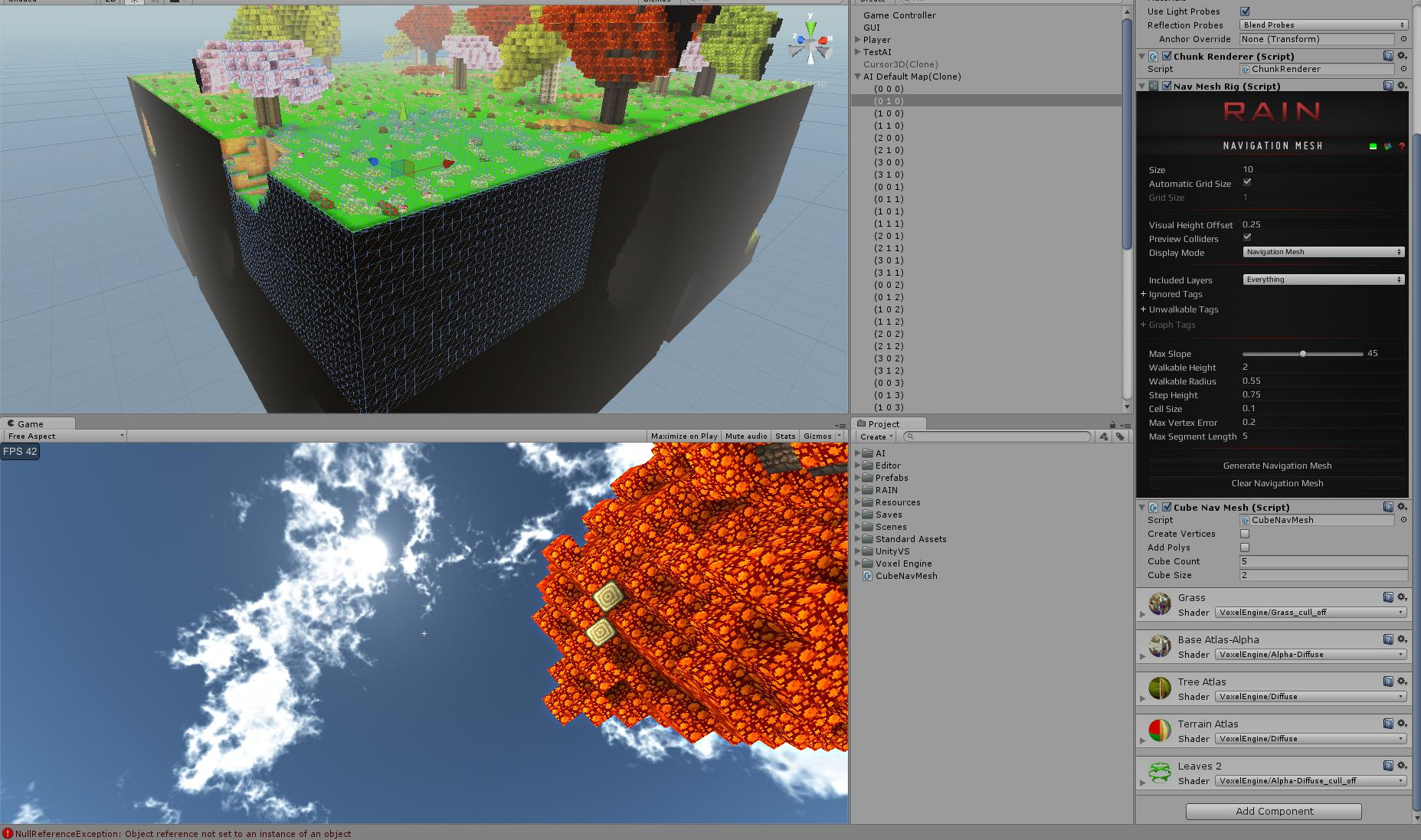The error is when trying to draw the navmesh graph

```NullReferenceException: Object reference not set to an instance of an object
RAINEditor.TypeEditors.RAINTypeEditor.DrawFieldForGizmo (GizmoType aType, RAIN.Serialization.FieldWalker aWalker)
RAINEditor.Core.RAINComponentEditor.OnGizmoGUI (RAIN.Core.RAINComponent aComponent, GizmoType aType)
UnityEditor.DockArea:OnGUI()```

I get this when manually adding CubeNavMesh to chunk during play (no cubemesh added previously)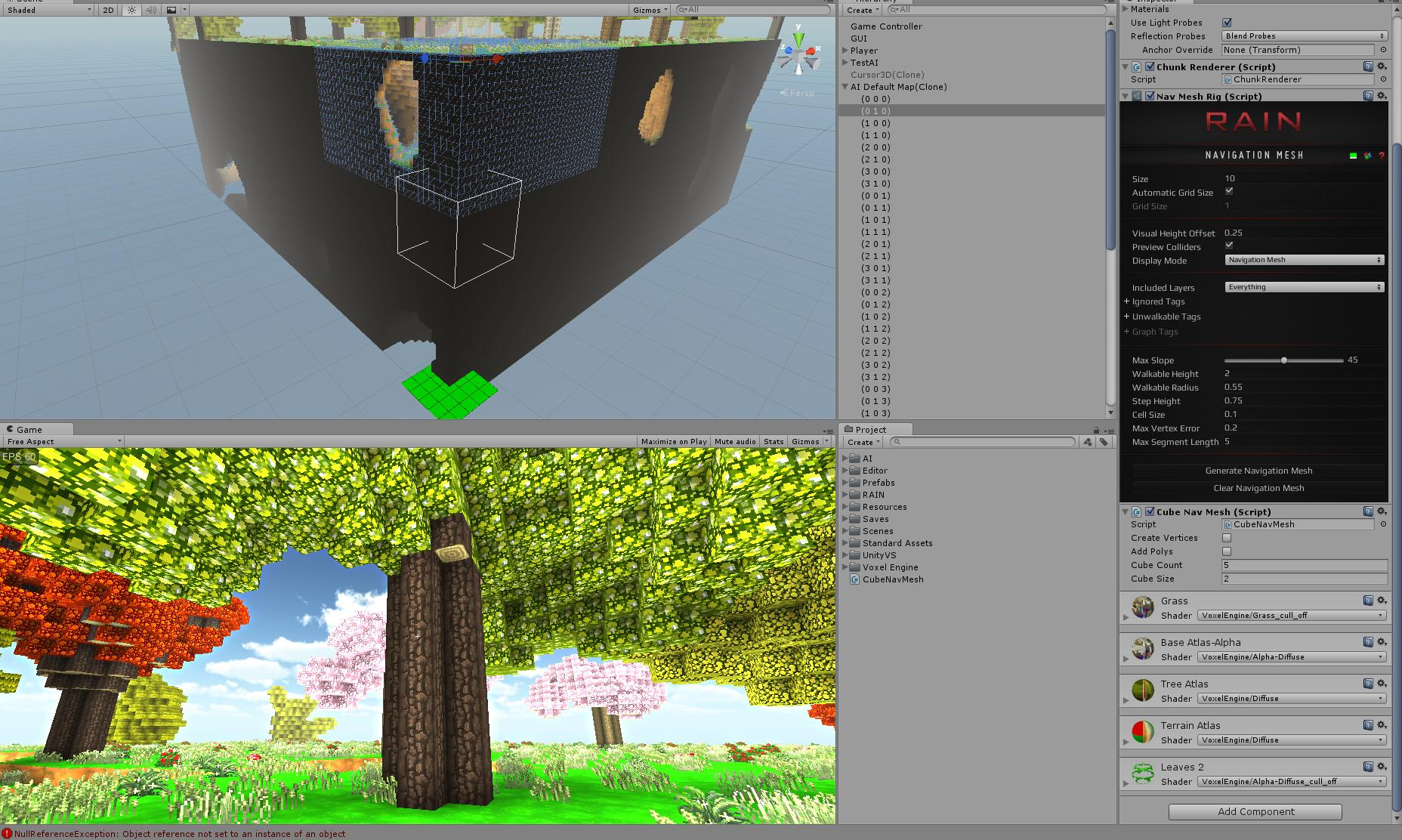The little detail i missed - This voxel terrain is runtime only.

#38921

@Sigil actually i can try to share the project with You - perhaps it will be a lot faster! You can get my contacts from Jester.

#38923

When CubeNavmesh has been added With AddComponent<Type>() and i try to create vertices and polys - same RAIN GUI error persists - nothing is displayed.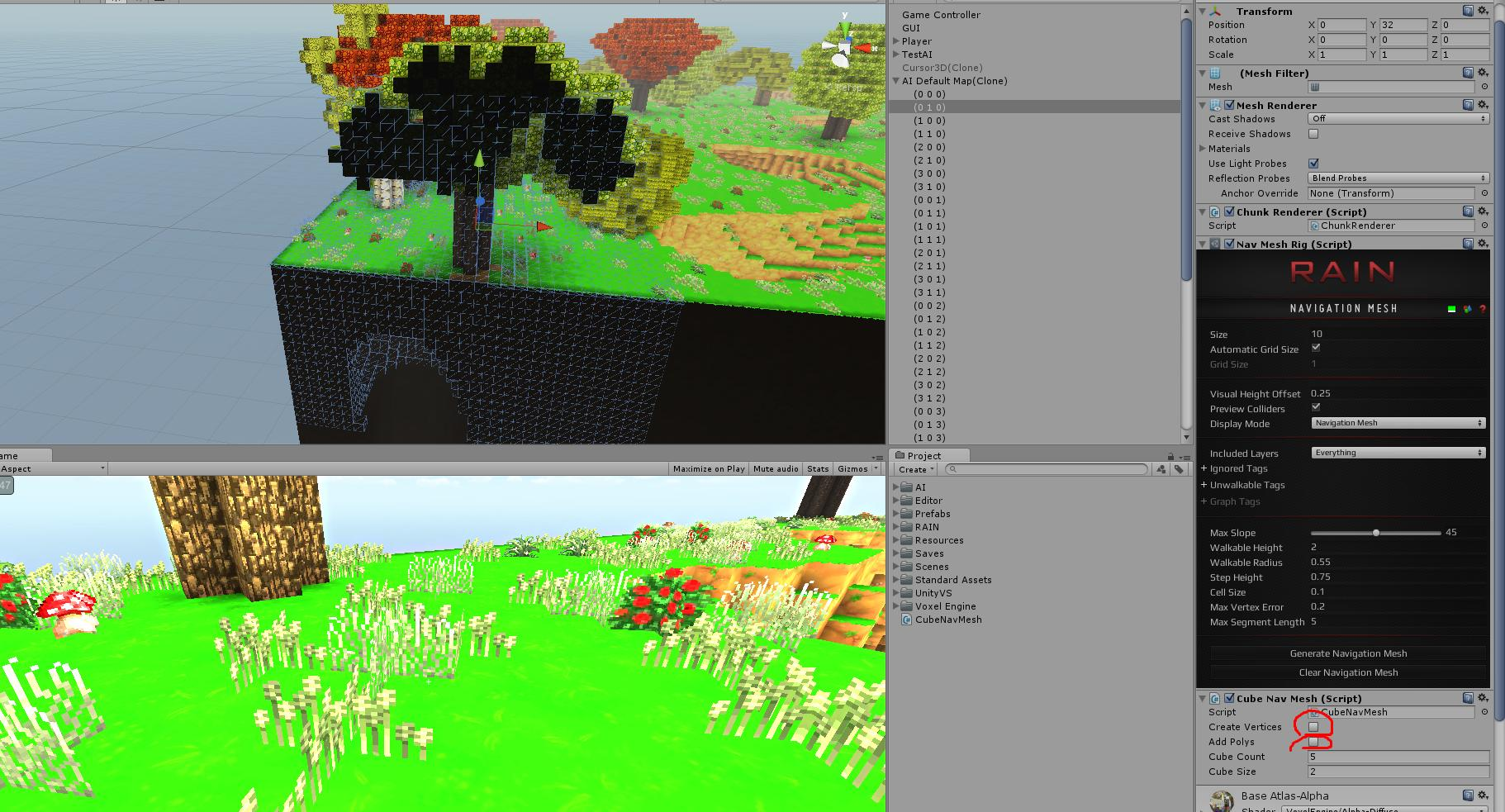#38928

Sorry @Sacristan, was pretty busy this weekend and wasn’t able to check on this. I will take another pass and try to improve some more on it. I know one of my next steps was to get it working properly with agent size, I am also curious about how hard this is going to hit memory wise.

Sharing the project may work as well, I will talk to Jester about it.

Viewing 14 posts - 1 through 14 (of 14 total)

You must be logged in to reply to this topic.